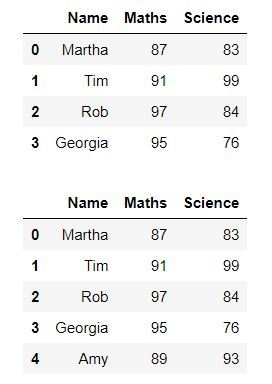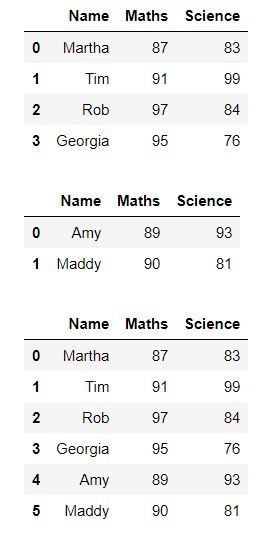GeeksforGeeks App
Open AppBrowser
Continue

# How to add one row in an existing Pandas DataFrame?

In this article, we’ll see how to add a new row of values to an existing dataframe. This can be used when we want to insert a new entry in our data that we might have missed adding earlier. There are different methods to achieve this. Now let’s see with the help of examples how we can do this

Example 1:

We can add a single row using DataFrame.loc. We can add the row at the last in our dataframe. We can get the number of rows using len(DataFrame.index) for determining the position at which we need to add the new row.

 `from` `IPython.display ``import` `display, HTML`` ` `import` `pandas as pd``from` `numpy.random ``import` `randint`` ` `dict` `=` `{``'Name'``:[``'Martha'``, ``'Tim'``, ``'Rob'``, ``'Georgia'``],``        ``'Maths'``:[``87``, ``91``, ``97``, ``95``],``        ``'Science'``:[``83``, ``99``, ``84``, ``76``]``       ``}`` ` `df ``=` `pd.DataFrame(``dict``)`` ` `display(df)`` ` `df.loc[``len``(df.index)] ``=` `[``'Amy'``, ``89``, ``93``] `` ` `display(df)`

Output:Example 2:

We can also add a new row using the DataFrame.append() function

 `from` `IPython.display ``import` `display, HTML`` ` `import` `pandas as pd``import` `numpy as np`` ` `dict` `=` `{``'Name'``:[``'Martha'``, ``'Tim'``, ``'Rob'``, ``'Georgia'``],``        ``'Maths'``:[``87``, ``91``, ``97``, ``95``],``        ``'Science'``:[``83``, ``99``, ``84``, ``76``]``       ``}`` ` `df ``=` `pd.DataFrame(``dict``)`` ` `display(df)`` ` `df2 ``=` `{``'Name'``: ``'Amy'``, ``'Maths'``: ``89``, ``'Science'``: ``93``}``df ``=` `df.append(df2, ignore_index ``=` `True``)`` ` `display(df)`

Output:Example 3:

We can also add multiple rows using the pandas.concat() by creating a new dataframe of all the rows that we need to add and then appending this dataframe to the original dataframe.

 `from` `IPython.display ``import` `display, HTML`` ` `import` `pandas as pd``import` `numpy as np`` ` `dict` `=` `{``'Name'``:[``'Martha'``, ``'Tim'``, ``'Rob'``, ``'Georgia'``],``        ``'Maths'``:[``87``, ``91``, ``97``, ``95``],``        ``'Science'``:[``83``, ``99``, ``84``, ``76``]``       ``}`` ` `df1 ``=` `pd.DataFrame(``dict``)``display(df1)`` ` `dict` `=` `{``'Name'``:[``'Amy'``, ``'Maddy'``],``        ``'Maths'``:[``89``, ``90``],``        ``'Science'``:[``93``, ``81``]``       ``}`` ` `df2 ``=` `pd.DataFrame(``dict``)``display(df2)`` ` `df3 ``=` `pd.concat([df1, df2], ignore_index ``=` `True``)``df3.reset_index()`` ` `display(df3)`

Output:My Personal Notes arrow_drop_up